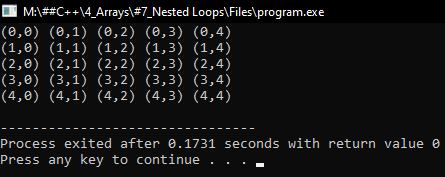# Nested Loops in C++

## Nested Loops in C++ with Examples:

In this article, I am going to discuss Nested Loops in C++ Language with examples. Please read our previous articles, where we discussed Binary Search in C++ with examples.

##### Nested Loops in C++:

Let us look at the nested ‘for’ loop. Usually, these are useful for accessing multi-dimensional arrays that are 2-D arrays or matrices. Commonly we write ‘for’ loop once. But now we are writing two ‘for’ loops, so let us write ‘for’ loop,

```for(int i = 0; i < 3; i++){
for(int j = 0; j < 3; j++){
cout << i << “ ” << j << endl;
}
}
```

So let us understand how this works and what are values will be displayed. When we say ‘cout << i << j’. ‘i’ and ‘j’ are two values initially ‘i’ is 0, 0 is less than 3. So, it will enter inside the 2nd ‘for’ loop. ‘j’ assigns 0, and j is less than 3. Here ‘i’ and ‘j’ are 0 and output will be ‘0 0’.Now it will go up ‘j++’. ‘i’ remains the same here and ‘j’ becomes 1 then ‘j’ is less than 3 so enter 2nd for loop again and print ‘i’ and ’j’. The output will be ‘0 1’.Next ‘j++’, ‘j’ becomes 2, and ‘i’ is still 0 and 2 is less than 3 so enter inside 2nd ‘for’ loop and print ‘i’ and ‘j’. So the output will be ‘0 2’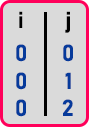Then ‘j++’ j becomes 3 and now ‘j’ is not less than 3, it is equal to 3. So, nothing is done so it will come out of this for loop and still it is inside the outer for loop. Now ‘i++’ so ‘I’ becomes 1, 1 is less than 3, so it will enter inside 2nd ‘for’ loop. Initially, again ‘j’ loop starts and ‘j’ becomes 0. The same steps will repeat here. The output will be:

‘1 0’

‘1 1’

‘1 2’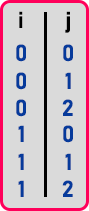Then again ‘i’ becomes 2, and it will enter the 2nd loop then ‘j’ will again initialize as 0 and previous steps will repeat here. So the final output is,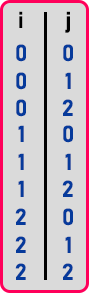So, these are the values printed so if you see how it has taken the values it is just like a two-dimensional array, i.e., 00 01 02 then 10 11 12 then 20 21 22.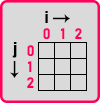In this way nested for loop will generate the indices for the 2D array. Here ‘i’ will act like a row index and ‘j’ will act like a column index. When we will write any application on matrices, we use nested for loops.

##### Program to print numbers using nested loop in C++:
```#include <iostream>
using namespace std;
int main()
{
for (int i = 0; i < 3; i++)
{
for (int j = 0; j < 3; j++)
{
cout << i << j << endl;
}
}
}
```
###### Output: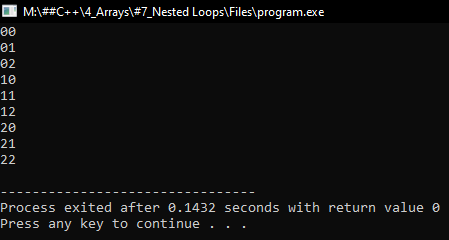##### Program to access the index of 2d Array:
```#include <iostream>
using namespace std;
int main()
{
for (int i = 0; i < 5; i++)
{
for (int j = 0; j < 5; j++)
{
cout << "(" << i << "," << j << ") ";
}
cout << endl;
}
}
```
###### Output: